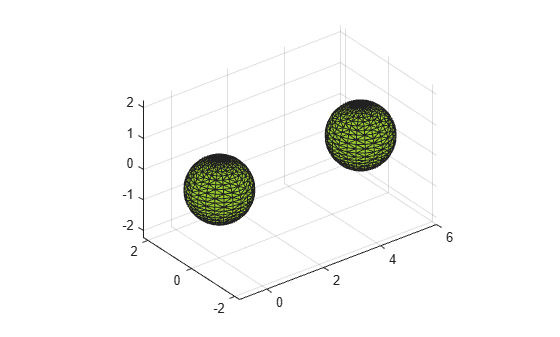# volume

Volume of 3-D alpha shape

## Syntax

``V = volume(shp)``
``V = volume(shp,RegionID)``

## Description

example

````V = volume(shp)` returns the volume of 3-D alpha shape `shp`.```

example

````V = volume(shp,RegionID)` returns the volume of a region of the alpha shape. `RegionID` is the ID for the region and `1` ≤ `RegionID` ≤ `numRegions(shp)`.```

## Examples

collapse all

Create a set of 3-D points.

```[x1, y1, z1] = sphere(24); x1 = x1(:); y1 = y1(:); z1 = z1(:); x2 = x1+5; P = [x1 y1 z1; x2 y1 z1]; P = unique(P,'rows');```

Create and plot an alpha shape using an alpha radius of 1.5.

```shp = alphaShape(P,1.5); plot(shp)```Compute the volume of the alpha shape.

`totalvol = volume(shp)`
```totalvol = 8.2468 ```

Compute the volumes of each of the two regions separately.

`regionvols = volume(shp, 1:numRegions(shp))`
```regionvols = 1×2 4.1234 4.1234 ```

## Input Arguments

collapse all

3-D alpha shape, specified as an `alphaShape` object. For more information, see `alphaShape`

Example: `shp = alphaShape(x,y,z)` creates a 3-D `alphaShape` object from the `(x,y,z)` point coordinates.

ID number for region in alpha shape, specified as a positive integer scalar between `1` and `numRegions(shp)`.

An alpha shape can contain several smaller regions, depending on the point set and parameters. Each of these smaller regions is assigned a unique `RegionID`, which numbers the regions from the largest area or volume to the smallest. For example, consider a 3-D alpha shape with two regions. The region with the largest volume has a `RegionID` of 1, and the smaller region has a `RegionID` of 2.

Example: `shp.RegionThreshold = area(shp,numRegions(shp)-2);` suppresses the two smallest regions in 2-D alpha shape `shp`.

Data Types: `double`

## Version History

Introduced in R2014b